# The calculation of the limit of a function

1. Using the continuity of the function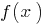, we try to substitute values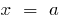into the function2.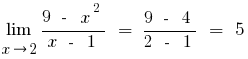3. If you calculate a limit at, then try in the numerator and the pronoun to make the brackets the highest degree of the unknown
4.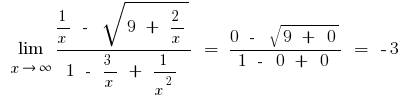5. If the result of the lookupreceived an expression of type,
6. a) try to decompose the numerator and the pronoun.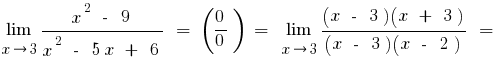b) if the numerator or denominator of the expression with square or cubic root, multiply the numerator and the denominator by the appropriate expression to get rid of the set of roots (sometimes administered to replace the expression root denotes the new variable)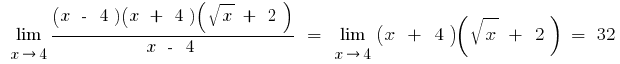C) if under the sign of the limit are trigonometric or inverse trigonometric functions, these boundaries reduce to the boundaries of the outstanding firstor its variations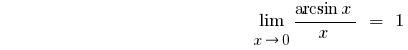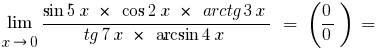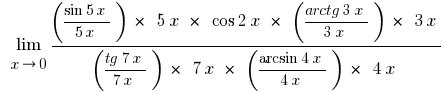Reducing the numerator and denominator variables that stand out, given that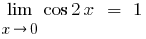, and given will persu outstanding border and its variations, we obtainTags:
Chapter:
Versions in other languages:
Share with friends: